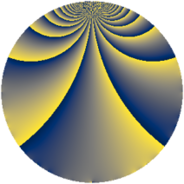# Properties

 Label 1805.2.eLevel $1805$ Weight $2$ Character orbit 1805.e Rep. character $\chi_{1805}(1151,\cdot)$ Character field $\Q(\zeta_{3})$ Dimension $224$ Sturm bound $380$

# Related objects

## Defining parameters

 Level: $$N$$ $$=$$ $$1805 = 5 \cdot 19^{2}$$ Weight: $$k$$ $$=$$ $$2$$ Character orbit: $$[\chi]$$ $$=$$ 1805.e (of order $$3$$ and degree $$2$$) Character conductor: $$\operatorname{cond}(\chi)$$ $$=$$ $$19$$ Character field: $$\Q(\zeta_{3})$$ Sturm bound: $$380$$

## Dimensions

The following table gives the dimensions of various subspaces of $$M_{2}(1805, [\chi])$$.

Total New Old
Modular forms 420 224 196
Cusp forms 340 224 116
Eisenstein series 80 0 80

## Trace form

 $$224 q + 2 q^{2} + 2 q^{3} - 110 q^{4} - 4 q^{6} + 12 q^{7} - 12 q^{8} - 114 q^{9} + O(q^{10})$$ $$224 q + 2 q^{2} + 2 q^{3} - 110 q^{4} - 4 q^{6} + 12 q^{7} - 12 q^{8} - 114 q^{9} + 8 q^{11} - 12 q^{12} - 6 q^{13} + 6 q^{14} + 4 q^{15} - 106 q^{16} + 6 q^{17} - 8 q^{18} - 8 q^{21} - 6 q^{22} + 6 q^{23} + 10 q^{24} - 112 q^{25} - 12 q^{26} - 16 q^{27} - 6 q^{29} - 32 q^{30} + 16 q^{31} + 24 q^{32} + 24 q^{33} - 10 q^{34} - 2 q^{35} - 118 q^{36} - 8 q^{37} - 36 q^{39} + 18 q^{40} - 16 q^{41} - 26 q^{42} - 4 q^{43} - 28 q^{44} + 8 q^{45} + 24 q^{46} + 12 q^{47} + 16 q^{48} + 256 q^{49} - 4 q^{50} + 4 q^{51} - 42 q^{52} - 4 q^{54} + 52 q^{56} + 52 q^{58} - 14 q^{59} + 6 q^{60} + 4 q^{61} + 36 q^{62} + 14 q^{63} + 136 q^{64} - 40 q^{65} + 14 q^{66} - 14 q^{67} - 100 q^{68} + 28 q^{69} - 8 q^{70} - 12 q^{71} + 28 q^{72} - 26 q^{73} + 42 q^{74} - 4 q^{75} + 28 q^{77} + 12 q^{78} - 2 q^{79} + 8 q^{80} - 132 q^{81} + 72 q^{82} - 36 q^{83} + 128 q^{84} - 8 q^{85} - 24 q^{86} - 44 q^{87} + 32 q^{88} - 18 q^{89} - 24 q^{90} - 12 q^{91} + 52 q^{92} + 28 q^{93} + 20 q^{94} - 124 q^{96} + 16 q^{97} + 32 q^{98} + 44 q^{99} + O(q^{100})$$

## Decomposition of $$S_{2}^{\mathrm{new}}(1805, [\chi])$$ into newform subspaces

The newforms in this space have not yet been added to the LMFDB.

## Decomposition of $$S_{2}^{\mathrm{old}}(1805, [\chi])$$ into lower level spaces

$$S_{2}^{\mathrm{old}}(1805, [\chi]) \cong$$ $$S_{2}^{\mathrm{new}}(95, [\chi])$$$$^{\oplus 2}$$$$\oplus$$$$S_{2}^{\mathrm{new}}(361, [\chi])$$$$^{\oplus 2}$$